Next: The Planck radiation law Up: Quantum statistics Previous: Maxwell-Boltzmann statistics

## Quantum statistics in the classical limit

The preceding analysis regarding the quantum statistics of ideal gases is summarized in the following statements. The mean number of particles occupying quantum state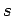is given by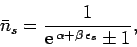(617)

where the upper sign corresponds to Fermi-Dirac statistics and the lower sign corresponds to Bose-Einstein statistics. The parameteris determined via(618)

Finally, the partition function of the gas is given by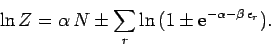(619)

Let us investigate the magnitude ofin some important limiting cases. Consider, first of all, the case of a gas at a given temperature when its concentration is made sufficiently low: i.e., whenis made sufficiently small. The relation (618) can only be satisfied if each term in the sum over states is made sufficiently small; i.e., if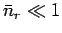or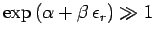for all states.

Consider, next, the case of a gas made up of a fixed number of particles when its temperature is made sufficiently large: i.e., when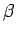is made sufficiently small. In the sum in Eq. (618), the terms of appreciable magnitude are those for which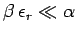. Thus, it follows that as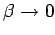an increasing number of terms with large values of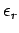contribute substantially to this sum. In order to prevent the sum from exceeding, the parametermust become large enough that each term is made sufficiently small: i.e., it is again necessary thatorfor all states.

The above discussion suggests that if the concentration of an ideal gas is made sufficiently low, or the temperature is made sufficiently high, thenmust become so large that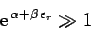(620)

for all. Equivalently, this means that the number of particles occupying each quantum state must become so small that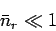(621)

for all. It is conventional to refer to the limit of sufficiently low concentration, or sufficiently high temperature, in which Eqs. (620) and Eqs. (621) are satisfied, as the classical limit.

According to Eqs. (617) and (620), both the Fermi-Dirac and Bose-Einstein distributions reduce to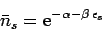(622)

in the classical limit, whereas the constraint (618) yields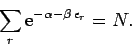(623)

The above expressions can be combined to give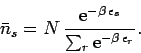(624)

It follows that in the classical limit of sufficiently low density, or sufficiently high temperature, the quantum distribution functions, whether Fermi-Dirac or Bose-Einstein, reduce to the Maxwell-Boltzmann distribution. It is easily demonstrated that the physical criterion for the validity of the classical approximation is that the mean separation between particles should be much greater than their mean de Broglie wavelengths.

Let us now consider the behaviour of the partition function (619) in the classical limit. We can expand the logarithm to give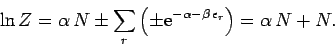(625)

However, according to Eq. (623),(626)

It follows that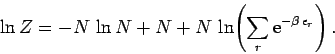(627)

Note that this does not equal the partition functioncomputed in Eq. (615) from Maxwell-Boltzmann statistics: i.e.,(628)

In fact,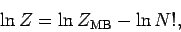(629)

or(630)

where use has been made of Stirling's approximation (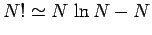), sinceis large. Here, the factorsimply corresponds to the number of different permutations of theparticles: permutations which are physically meaningless when the particles are identical. Recall, that we had to introduce precisely this factor, in an ad hoc fashion, in Sect. 7.7 in order to avoid the non-physical consequences of the Gibb's paradox. Clearly, there is no Gibb's paradox when an ideal gas is treated properly via quantum mechanics.

In the classical limit, a full quantum mechanical analysis of an ideal gas reproduces the results obtained in Sects. 7.6 and 7.7, except that the arbitrary parameter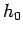is replaced by Planck's constant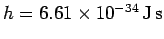.

A gas in the classical limit, where the typical de Broglie wavelength of the constituent particles is much smaller than the typical inter-particle spacing, is said to be non-degenerate. In the opposite limit, where the concentration and temperature are such that the typical de Broglie wavelength becomes comparable with the typical inter-particle spacing, and the actual Fermi-Dirac or Bose-Einstein distributions must be employed, the gas is said to be degenerate.Next: The Planck radiation law Up: Quantum statistics Previous: Maxwell-Boltzmann statistics
Richard Fitzpatrick 2006-02-02